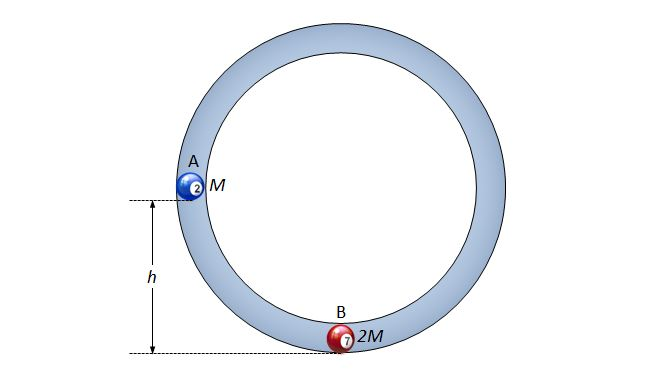Moving in a curved surfaceObject $A$ with a mass of $M$ is about to slide down a curved surface from the height of $h$ to collide with object $B$ at the bottom with a mass of $2M,$ as shown above. If the coefficient of restitution between the two objects is $0.5,$ then what is the speed of object $B$ immediately after the collision? (Ignore air resistance, all frictional forces and the sizes of the objects. $g$ denotes gravitational acceleration.)

×